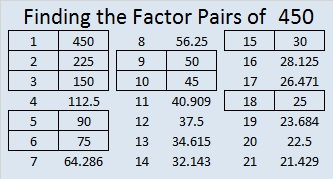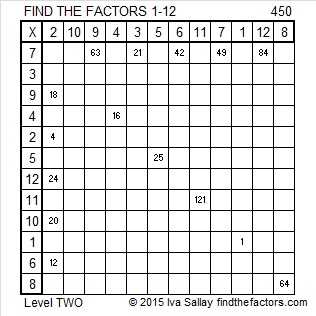# How to Simplify √450

What’s easier – dividing a number by 2 twice or dividing by 4 once? Most people would agree that dividing by a single digit number like 9 one time is easier than dividing by 3 two times. It also cuts the chance of making a mistake in half.

To simplify square roots, I’ve modified the cake method to look for some specific SQUARE factors rather than beginning with ALL of its prime factors.

Divisibility tricks let me know which square factors to try. I always divide out easy and very common perfect squares 100, 4, 9, and 25 first. (About 82% of reducible square roots are divisible by 4 and/or 9.) Once any of those that apply have been divided out, I look to see if 6 or 10 can be divided out because its easier to divide by 6 or 10 once than to divide by 2 and then by 3 or 5. Only after I have divided out those very easy divisors will I look to divide out any remaining prime factors 2, 3, 5, 7, 11, and so forth. Let me demonstrate this method to simplify √450.

• 450 doesn’t end in 00, so it’s not divisible by 100.
• The last two digits, 50, are not divisible by 4, so 450 is not divisible by 4.
• 4 + 5 + 0 = 9, so 450 is divisible by 9. Therefore, I do a simple division problem, 450 ÷ 9 = 50, leaving room on the page to do any other needed division problems above it.
• The previous quotient, 50, is not divisible by 9, but any number ending in 25, 50, or 75 is divisible by 25, so I divide it by 25 and get 2. Now I am finished dividing.
• The numbers on the outside of the cake are 9, 25, and 2. I take the square root of each of those numbers and get 3, 5, and √2. The product of those square roots is 15√2. Thus √450 = 15√2.Most people have been taught to use a factor tree to find square roots. This is probably 450’s most common factor tree:I like how much more compact and clear this modified cake method is instead. It may take some practice to get used to it, but I will show more examples of it in the future to make it more familiar.

The following puzzle doesn’t have anything to do with the number 450 except it’s the number I gave it to distinguish it from every other puzzle I make. I put the factors of 450 immediately after the puzzle to separate the puzzle from its solution that I add to the post the next day.Print the puzzles or type the factors on this excel file:  12 Factors 2015-04-06

——————————————————————————————————————-

• 450 is a composite number.
• Prime factorization: 450 = 2 x 3 x 3 x 5 x 5, which can be written 450 = 2 x (3^2) x (5^2)
• The exponents in the prime factorization are 1, 2 and 2. Adding one to each and multiplying we get (1 + 1)(2 + 1)(2 + 1) = 2 x 3 x 3 = 18. Therefore 450 has exactly 18 factors.
• Factors of 450: 1, 2, 3, 5, 6, 9, 10, 15, 18, 25, 30, 45, 50, 75, 90, 150, 225, 450
• Factor pairs: 450 = 1 x 450, 2 x 225, 3 x 150, 5 x 90, 6 x 75, 9 x 50, 10 x 45, 15 x 30 or 18 x 25
• Taking the factor pair with the largest square number factor, we get √450 = (√225)(√2) = 15√2 ≈ 21.2132——————————————————————————————————————-